# Review — Word2Vec: Efficient Estimation of Word Representations in Vector Space

## Word2Vec: Using CBOW or Skip-Gram to Convert Words to Meaningful Vectors, i.e. Word Representation Learning

In this story, Efficient Estimation of Word Representations in Vector Space, (Word2Vec), by Google, is reviewed. In this paper:

• Word2Vec is proposed to convert words into vectors that have semantic and syntactic meanings, i.e. word representations, e.g.: vector(”King”)-vector(”Man”)+vector(”Woman”) results , we can obtain vector(”Queen”).
• Two approaches are proposed for Word2Vec: CBOW (Continuous Bag-of-Words) predicts missing word from its previous and future words while Skip-Gram predicts missing words that surrounding the current word.
• By doing so, a language model is built, which is useful for other tasks such as machine translation, image captioning, etc.

This is a paper in 2013 ICLR with over 24000 citations. (Sik-Ho Tsang @ Medium) As we can see, training samples can be obtained by removing words in a complete sentence, and predicting them back again. This is a kind of useful concept in self-supervised learning.

# Outline

1. CBOW (Continuous Bag-of-Words) Model
2. Skip-Gram Model
3. Experimental Results

# 1. CBOW (Continuous Bag-of-Words) Model

• Assume we have a corpus which has a vocabulary of V words, a context of C words.
• At the input layer, each word is encoded using 1-of-V coding.
• A dense representation of N-dimensional word vector, an embedding/projection matrix W of dimensions V×N at the input and a context matrix W of dimensions N×V at the output.
• CBOW take words surrounding a given word and try to predict the missing one at the middle.
• Via the embedding/projection matrix, a N-dimensional vector is obtained which is the average of C word vectors.
• From this vector, the probabilities for each word is computed in the vocabulary. Word with highest probability is the predicted word.

# 2. Skip-Gram Model

• Different from CBOW, Skip-Gram taked one word and try to predict words that occur around it.
• At the output, we try to predict C different words.
• (There is no mathematical equations for the cost function in the original paper. In another paper from the same authors, which is the follow up work of this paper, “Distributed Representations of Words and Phrases and their Compositionality”, Skip-Gram model cost function is mentioned.)
• The objective of the Skip-Gram model is to maximize the average log probability:
• where T is the number of sentences to be predicted.
• The probability is the Softmax function.
• where W is the vocabulary size (i.e. V in this paper).
• This objective of CBOW is similar to this one.

The computation complexity for the denominator is large when the vocabulary size is large.

# 3. Experimental Results

## 3.1. Evaluation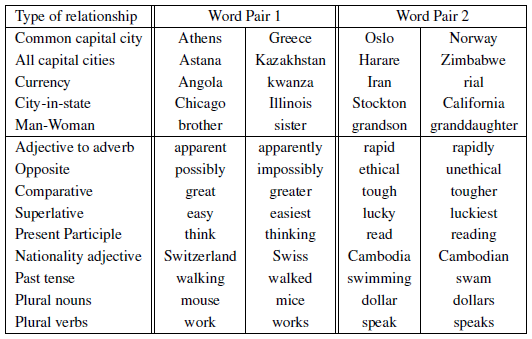Examples of five types of semantic and nine types of syntactic questions in the Semantic-Syntactic Word Relationship test set
• Overall, there are 8869 semantic and 10675 syntactic questions.
• The questions in each category were created in two steps: first, a list of similar word pairs was created manually. Then, a large list of questions is formed by connecting two word pairs.
• For example, a list of 68 large American cities and the states is made, and formed about 2.5K questions by picking two word pairs at random.

## 3.2. Dataset

• A Google News corpus is used for training the word vectors. This corpus contains about 6B tokens. The vocabulary size is restricted to 1 million most frequent words.
• For choosing the best models, models are trained on subsets of the training data, with vocabulary restricted to the most frequent 30k words.

## 3.3. CBOW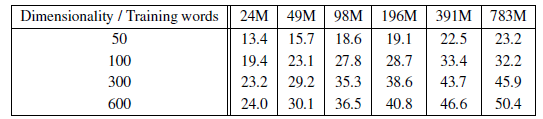Accuracy on subset of the Semantic-Syntactic Word Relationship test set, using word vectors from the CBOW architecture with limited vocabulary
• It can be seen that after some point, adding more dimensions or adding more training data provides diminishing improvements.
• It is better to increase both vector dimensionality and the amount of the training data together.

## 3.4. SOTA Comparison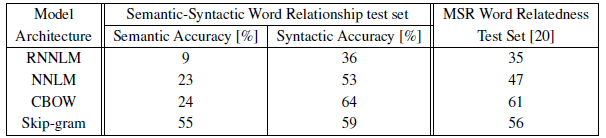Comparison of architectures using models trained on the same data, with 640-dimensional word vectors with limited vocabulary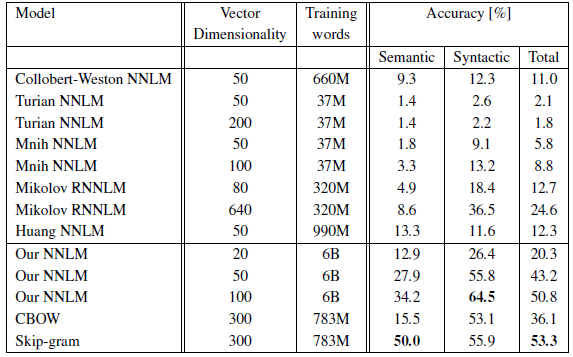Comparison of publicly available word vectors on the Semantic-Syntactic Word Relationship test set with full vocabulary
• The CBOW architecture works better than the NNLM on the syntactic tasks, and about the same on the semantic one.

The Skip-gram architecture works slightly worse on the syntactic task than the CBOW model (but still better than the NNLM), and much better on the semantic part of the test than all the other models.

## 3.5. Data vs Epoch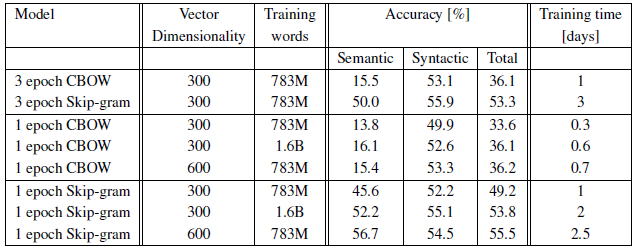Comparison of models trained for three epochs on the same data and models trained for one epoch
• Training a model on twice as much data using one epoch gives comparable or better results than iterating over the same data for three epochs.

## 3.6. Large Scale Parallel Training of Models

• DistBelief is the framework using replicas for CPU multitasking.
• 50 to 100 model replicas are used during the training. The number of CPU cores is an estimate since the data center machines are shared with other production tasks.
• The CPU usage of the CBOW model and the Skip-Gram model are much closer to each other than their single-machine one.

## 3.7. Microsoft Research Sentence Completion Challenge

• This task consists of 1040 sentences, where one word is missing in each sentence and the goal is to select word that is the most coherent with the rest of the sentence, given a list of five reasonable choices.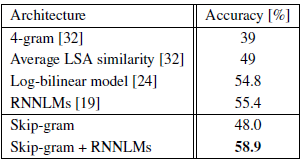Comparison and combination of models on the Microsoft Sentence Completion Challenge
• Skip-gram 640-dimensional model is trained on 50M words.
• While the Skip-gram model itself does not perform on this task better than LSA similarity, the scores from this model are complementary to scores obtained with RNNLMs, and a weighted combination leads to a new state of the art result 58.9% accuracy (59.2% on the development part of the set and 58.7% on the test part of the set).

## 3.8. Examples of the Learned Relationships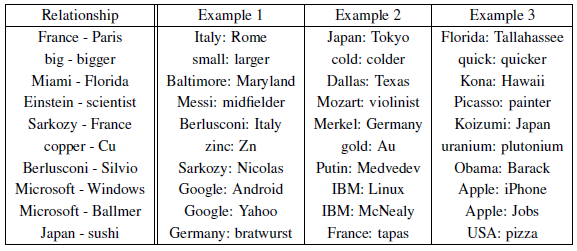Examples of the word pair relationships, using the best word vectors

## Reference

[2013 ICLR] [Word2Vec]
Efficient Estimation of Word Representations in Vector Space

## Natural Language Processing (NLP)

Language Model: 2007 [Bengio TNN’07] 2013 [Word2Vec]
Machine Translation: 2014
[Seq2Seq] [RNN Encoder-Decoder] 2015 [Attention Decoder/RNNSearch]
Image Captioning: 2015 [m-RNN] [R-CNN+BRNN] [Show and Tell/NIC]

--

--